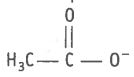Nucleophiles and electrophiles are reaction intermediates having electron rich and electron deficient centres respectively. Hence, they tend to attack electron deficient and electron rich centres respectively. Classify the following species as electrophile and nucleophiles.

1.  ${\mathrm{H}}_{3}{\mathrm{CO}}^{-}$

2.3.  $\mathrm{Cl}$

4.  ${\mathrm{Cl}}_{2}\mathrm{C}:$

5. ${\left({\mathrm{H}}_{3}\mathrm{C}\right)}_{3}{\mathrm{C}}^{+}$

6. ${\mathrm{Br}}^{-}$

7.  ${\mathrm{H}}_{3}\mathrm{COH}$

8.  $\mathrm{R}-\mathrm{NH}-\mathrm{R}$

Electrophiles are electron deficient species. They may be natural or positively charged e.g., (iii) $\mathrm{Cl}$, (iv) ${\mathrm{Cl}}_{2}\mathrm{C}:$, (v) ${\left({\mathrm{H}}_{3}\mathrm{C}\right)}_{3}{\mathrm{C}}^{+}$
Nucleophiles are electron rich species. They may be neutral or negative charge e.g.,

(i) ${\mathrm{H}}_{3}{\mathrm{CO}}^{-}$, (ii), (vi) ${\mathrm{Br}}^{-}$, (vii) ${\mathrm{H}}_{3}\mathrm{COH}$, (viii) $\mathrm{R}-\mathrm{NH}-\mathrm{R}$